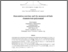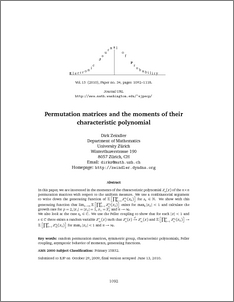# Permutation matrices and the moments of their characteristics polynomials

Zeindler, Dirk (2010) Permutation matrices and the moments of their characteristics polynomials. Electronic Journal of Probability, 15. pp. 1092-1118.Preview
PDF (781-2659-1-PB)
781_2659_1_PB.pdf - Published Version

## Abstract

In this paper, we are interested in the moments of the characteristic polynomial Zn(x) of the n×n permutation matrices with respect to the uniform measure. We use a combinatorial argument to write down the generating function of E[∏pk=1Zskn(xk)] for sk∈N. We show with this generating function that limn→∞E[∏pk=1Zskn(xk)] exists exists for maxk|xk|<1 and calculate the growth rate for p=2, |x1|=|x2|=1, x1=x2 and n→∞. We also look at the case sk∈C. We use the Feller coupling to show that for each |x|<1 and s∈C there exists a random variable Zs∞(x) such that Zsn(x)→dZs∞(x) and E[∏pk=1Zskn(xk)]→E[∏pk=1Zsk∞(xk)] for maxk|xk|<1 and n→∞.

Item Type:
Journal Article
Journal or Publication Title:
Electronic Journal of Probability
Uncontrolled Keywords:
/dk/atira/pure/subjectarea/asjc/1800/1804
Subjects:
Departments:
ID Code:
70750
Deposited By:
Deposited On:
12 Sep 2014 08:08
Refereed?:
Yes
Published?:
Published